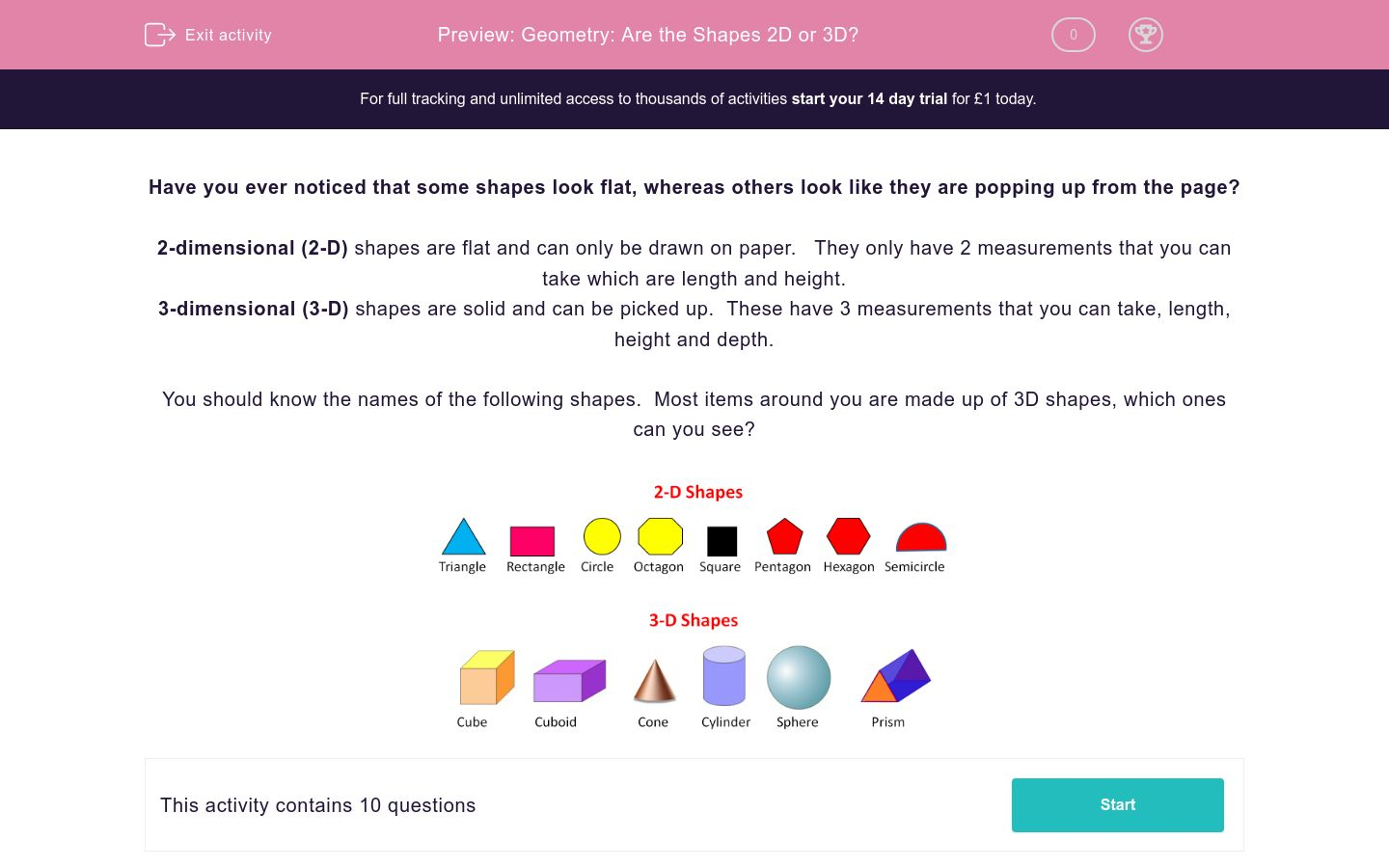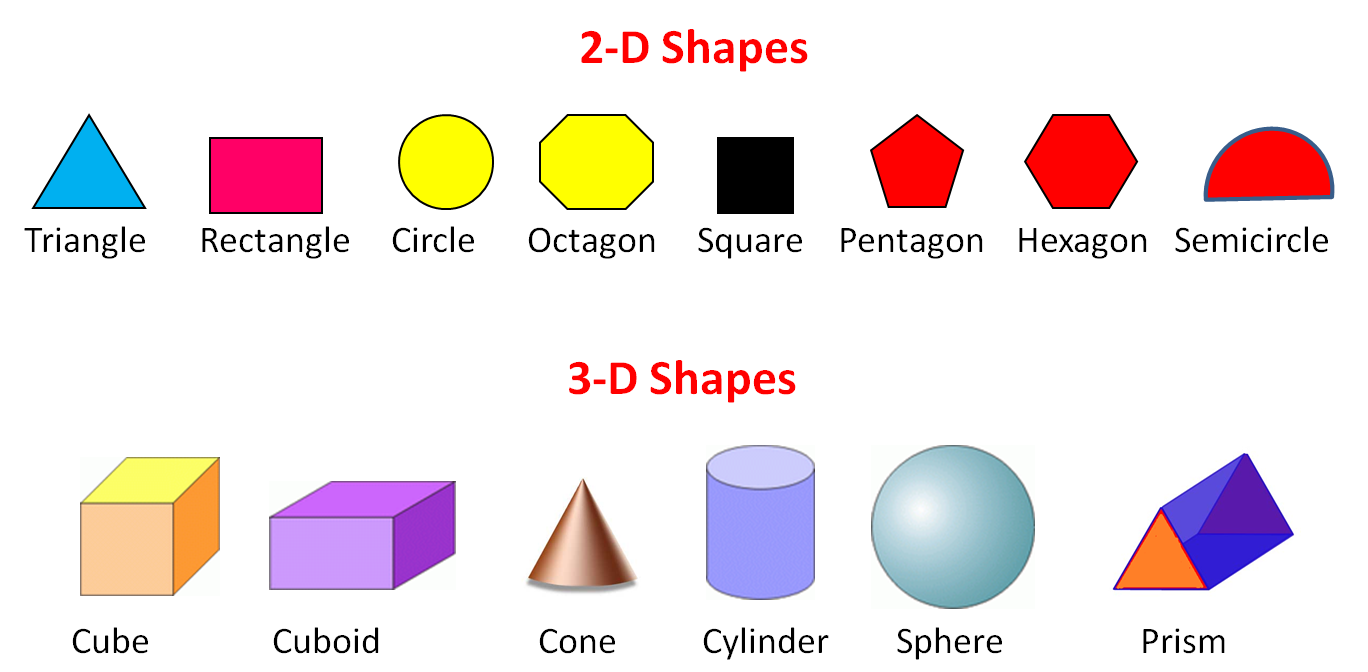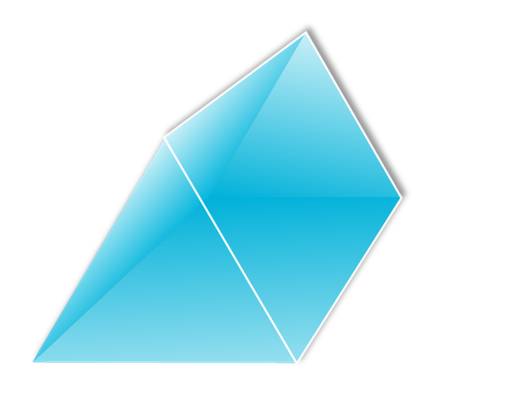# Geometry: Are the Shapes 2D or 3D?

In this worksheets, students state whether a given shape is 2D or 3D.Key stage:  KS 2

Curriculum topic:   Maths and Numerical Reasoning

Curriculum subtopic:   3D Shapes

Difficulty level:### QUESTION 1 of 10

Have you ever noticed that some shapes look flat, whereas others look like they are popping up from the page?

2-dimensional (2-D) shapes are flat and can only be drawn on paper.   They only have 2 measurements that you can take which are length and height.

3-dimensional (3-D) shapes are solid and can be picked up.  These have 3 measurements that you can take, length, height and depth.

You should know the names of the following shapes.  Most items around you are made up of 3D shapes, which ones can you see?Is a    CIRCLE  2-dimensional or 3-dimensional shape?

2-D

3-D

Can you identify the 3D shape below?Cube

Circle

Sphere

Cone

Can you correctly categorise the shapes below?

Cube

Circle

Sphere

Cone

True or false, a  CUBOID is a 2-dimensional shape?

True

False

Can you categorise the shapes in the list below, are they 2D or 3D?

 2D Shape 3D Shape Cube Sphere Rectangle Cone Cylinder Square Circle Prism

Can you complete the sentence below about a 3D shape?

 2D Shape 3D Shape Cube Sphere Rectangle Cone Cylinder Square Circle Prism

Complete the 2 questions below about an OCTAGON.

 2D Shape 3D Shape Cube Sphere Rectangle Cone Cylinder Square Circle Prism

Can you give the name of the shape below if it was in 2D?Can you label the shape below?Rectangular Prism

Triangular Prism

Hexagonal Prism

Triangle

Classify and name the 3D Shape below.Rectangular Prism

Triangular Prism

Hexagonal Prism

Triangle

• Question 1

Is a    CIRCLE  2-dimensional or 3-dimensional shape?

2-D
EDDIE SAYS
A circle is a 2D shape. If you were to make a circle 2D it is called a sphere. Remember a 2D shape is much easier to draw than a 3D shape, this is a way to check which category it belongs to.
• Question 2

Can you identify the 3D shape below?Sphere
EDDIE SAYS
This is the 3D version of a circle and it is called a sphere! (Quite a hard word to pronounce correctly!) What sphere's can you see around you right now?
• Question 3

Can you correctly categorise the shapes below?

EDDIE SAYS
How did you do? The only 3D shape was a cylinder. A cylinder is a common 3D shape, it could also be described as a tube. This is a good example of a cylinder. Remember apply the rule - if you can draw it in a simple way, it's probably a 2D shape.
• Question 4

True or false, a  CUBOID is a 2-dimensional shape?

False
EDDIE SAYS
The answer here is false! A cuboid is a 3D shape as it has height, length and depth that can be measured. Cuboids make up many storage containers in your house. Have a look around, can you see any cuboids from where you are?
• Question 5

Can you categorise the shapes in the list below, are they 2D or 3D?

 2D Shape 3D Shape Cube Sphere Rectangle Cone Cylinder Square Circle Prism
EDDIE SAYS
How are you doing? If you are not sure here, always look back to the introduction. It's important that you know the difference between 2D and 3D at this stage. Go on a shape hunt at home, and have a go at labelling household items until you are confident!
• Question 6

Can you complete the sentence below about a 3D shape?

EDDIE SAYS
Remember the difference between 2D and 3D in terms of measurement is that a 2D has two things you can measure (2 for 2D) and a 3D shape has 3! Have you seen the pattern here?
• Question 7

Complete the 2 questions below about an OCTAGON.

EDDIE SAYS
Did you answer in the way we suggested? It's really important to read questions carefully. Did you know that the name of a shape often gives a clue as to the number of sides that shape has? For example: Pent = 5 Hex = 6 Hept = 7 Oct = 8? Clever isn't it?
• Question 8

Can you give the name of the shape below if it was in 2D?semi circle
semi-circle
EDDIE SAYS
This is half of a sphere, but we know that a sphere is a 3D shape. So we need the 2D answer. The shape would be half of a circle and in maths terms, we call this a semi-circle. If you worked this out, well done!
• Question 9

Can you label the shape below?Triangular Prism
EDDIE SAYS
Hopefully, you can see from the complexity of this shape it is 3D! That means we can automatically discount the word 'triangle'. It is definitely a type of prism, so we need to look at the end and see what shape runs through this prism? Can you see the triangle at the end? This means that we've found the answer. It a triangular prism! Looks like a very famous Swiss chocolate bar!
• Question 10

Classify and name the 3D Shape below.EDDIE SAYS
The party hat is an example of a 3D cone, remember that next time you wear one!
---- OR ----

Sign up for a £1 trial so you can track and measure your child's progress on this activity.

### What is EdPlace?

We're your National Curriculum aligned online education content provider helping each child succeed in English, maths and science from year 1 to GCSE. With an EdPlace account you’ll be able to track and measure progress, helping each child achieve their best. We build confidence and attainment by personalising each child’s learning at a level that suits them.

Get started